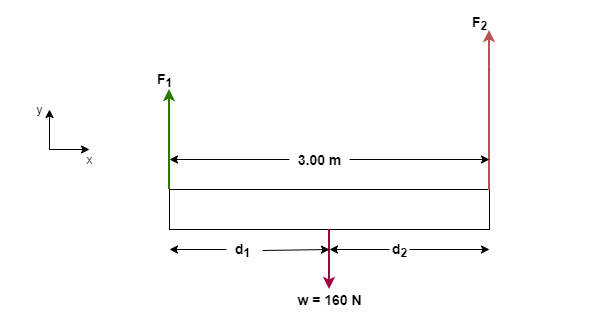# Problem: Two people are carrying a uniform wooden board that is 3.00 m long and weighs 160 N. If one person applies an upward force equal to 60 N at one end, at what point does the other person lift? Begin with a free body diagram of the board.

###### FREE Expert Solution

The free-body diagram of the board is as shown in the figure below.Torque:

$\overline{){\mathbf{\tau }}{\mathbf{=}}{\mathbf{F}}{\mathbf{d}}}$

Force applied by one person on one end is F1 = 60N.

Since the weight of the board is 160N, the force applied by the other person on the other end is F2 = 160 - 60 = 100 N

Both forces must apply torque at the center of the bar, which balances each other.

99% (333 ratings)###### Problem Details

Two people are carrying a uniform wooden board that is 3.00 m long and weighs 160 N. If one person applies an upward force equal to 60 N at one end, at what point does the other person lift?

Begin with a free body diagram of the board.